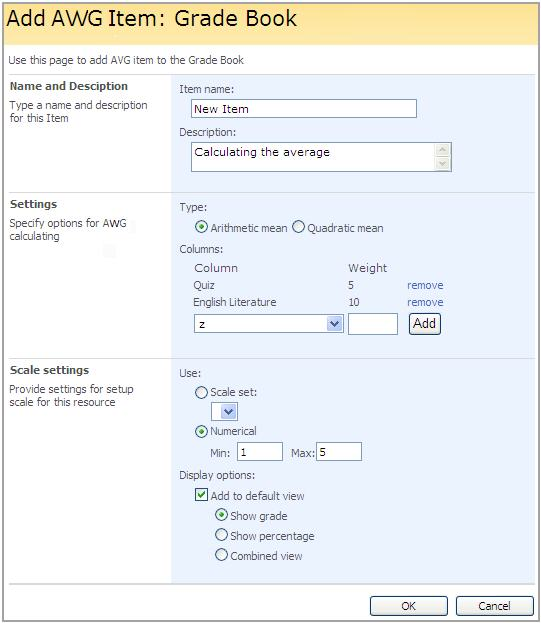﻿

The option allows calculating the average of several Grade Book columns of a learner.

To perform the action,

1. Enter the Grade Book section

2. Click Settings

4. The form for adding item will appear:

Fill in the fields as required:

- Name – enter a title of a column

- Description – enter a short description

- Settings:

Ø Type – specify whether you want the system to display the arithmetic (select Arithmetic mean) or the quadratic (select Quadratic mean) mean.

Ø Columns – select a column from the drop down list and press Add. To delete the column, click Remove. Note that two or more columns should be selected.

Ø Weight – enter any positive number. The number is a coefficient which denotes the importance of a column. The higher the number the more important a column.

 NOTE When calculating the average for a SCORM package points are used instead of percentage. Make sure the value specified in the Weight column is small.

The calculation is done according to the following formulae:

Arithmetic mean: Vavg = SUM (V1 * M1… Vn * Mn) / SUM (M1... Mn)

Quadratic mean: Vavg2 = SQRT (SUM (V1^2 * M1... Vn^2 * Mn) / SUM (M1... Mn)), where V is the score (from 0 to 100%) – a mark from a column

M is the coefficient specified in the Weight field.

- Scale Settings:

Ø Use – specify the scale you want to use for the resource. To set your own scale, fill in the Numerical option fields.

Ø Display options – specify whether you want the result of calculation to be displayed in the Default view and the way you want it to be displayed (in Grades, Percentage or as the Combined view)Fig. Calculating AWG

5. Click OK to save setting. Click Cancel to discard changes.